Data encryption standard (DES) | Set 1

Data encryption standard (DES) has been found vulnerable against very powerful attacks and therefore, the popularity of DES has been found slightly on decline.

DES is a block cipher, and encrypts data in blocks of size of 64 bit each, means 64 bits of plain text goes as the input to DES, which produces 64 bits of cipher text. The same algorithm and key are used for encryption and decryption, with minor differences. The key length is 56 bits. The basic idea is show in figure.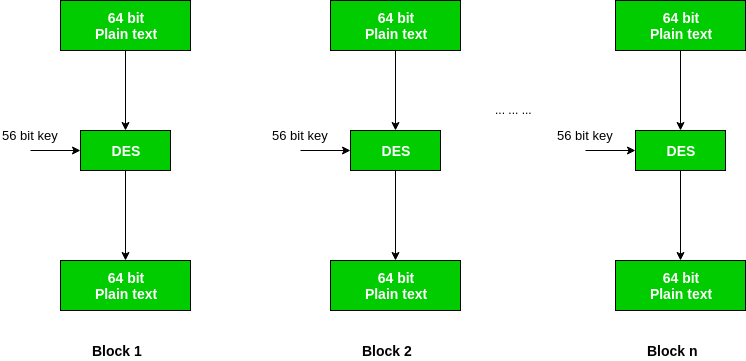We have mention that DES uses a 56 bit key. Actually, the initial key consists of 64 bits. However, before the DES process even starts, every 8th bit of the key is discarded to produce a 56 bit key. That is bit position 8, 16, 24, 32, 40, 48, 56 and 64 are discarded.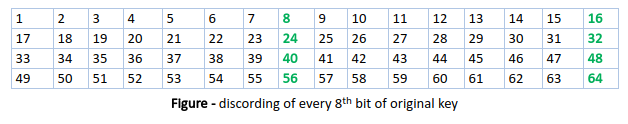Thus, the discarding of every 8th bit of the key produces a 56-bit key from the original 64-bit key.

DES is based on the two fundamental attributes of cryptography: substitution (also called as confusion) and transposition (also called as diffusion). DES consists of 16 steps, each of which is called as a round. Each round performs the steps of substitution and transposition. Let us now discuss the broad-level steps in DES.

1. In the first step, the 64 bit plain text block is handed over to an initial Permutation (IP) function.
2. The initial permutation performed on plain text.
3. Next the initial permutation (IP) produces two halves of the permuted block; says Left Plain Text (LPT) and Right Plain Text (RPT).
4. Now each LPT and RPT to go through 16 rounds of encryption process.
5. In the end, LPT and RPT are rejoined and a Final Permutation (FP) is performed on the combined block
6. The result of this process produces 64 bit cipher text.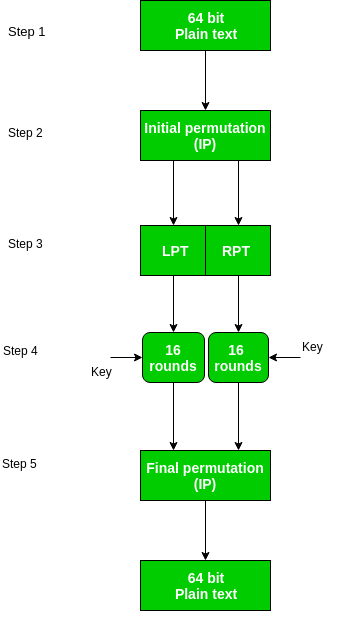Initial Permutation (IP) –
As we have noted, the Initial permutation (IP) happens only once and it happens before the first round. It suggests how the transposition in IP should proceed, as show in figure.

For example, it says that the IP replaces the first bit of the original plain text block with the 58th bit of the original plain text, the second bit with the 50th bit of the original plain text block and so on.

This is nothing but jugglery of bit positions of the original plain text block. the same rule applies for all the other bit positions which shows in the figure.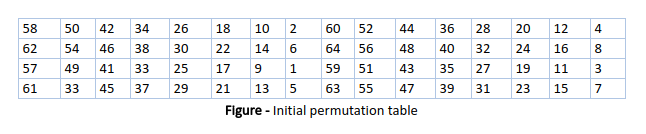As we have noted after IP done, the resulting 64-bit permuted text block is divided into two half blocks. Each half block consists of 32 bits, and each of the 16 rounds, in turn, consists of the broad level steps outlined in figure.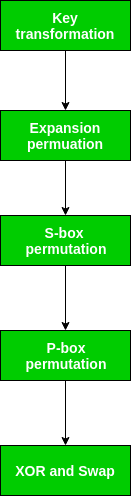Step-1: Key transformation –
We have noted initial 64-bit key is transformed into a 56-bit key by discarding every 8th bit of the initial key. Thus, for each a 56-bit key is available. From this 56-bit key, a different 48-bit Sub Key is generated during each round using a process called as key transformation. For this the 56 bit key is divided into two halves, each of 28 bits. These halves are circularly shifted left by one or two positions, depending on the round.

For example, if the round number 1, 2, 9 or 16 the shift is done by only position for other rounds, the circular shift is done by two positions. The number of key bits shifted per round is show in figure.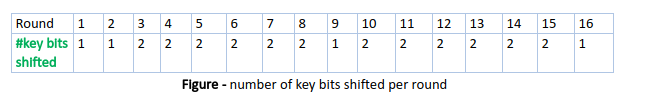After an appropriate shift, 48 of the 56 bit are selected. for selecting 48 of the 56 bits the table show in figure given below. For instance, after the shift, bit number 14 moves on the first position, bit number 17 moves on the second position and so on. If we observe the table carefully, we will realize that it contains only 48 bit positions. Bit number 18 is discarded (we will not find it in the table), like 7 others, to reduce a 56-bit key to a 48-bit key. Since the key transformation process involves permutation as well as selection of a 48-bit sub set of the original 56-bit key it is called Compression Permutation.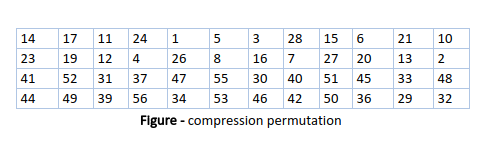Because of this compression permutation technique, a different subset of key bits is used in each round. That’s make DES not easy to crack.

Step-2: Expansion Permutation –
Recall that after initial permutation, we had two 32-bit plain text areas called as Left Plain Text(LPT) and Right Plain Text(RPT). During the expansion permutation, the RPT is expanded from 32 bits to 48 bits. Bits are permuted as well hence called as expansion permutation. This happens as the 32 bit RPT is divided into 8 blocks, with each block consisting of 4 bits. Then, each 4 bit block of the previous step is then expanded to a corresponding 6 bit block, i.e., per 4 bit block, 2 more bits are added.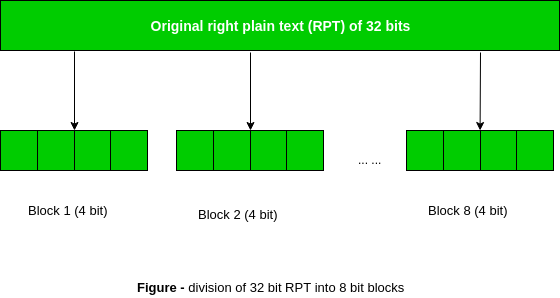This process results into expansion as well as permutation of the input bit while creating output. Key transformation process compresses the 56-bit key to 48 bits. Then the expansion permutation process expands the 32-bit RPT to 48-bits. Now the 48-bit key is XOR with 48-bit RPT and resulting output is given to the next step, which is the S-Box substitution.

 #include using namespace std; string hex2bin(string s){         // hexadecimal to binary conversion     unordered_map mp;     mp['0']= "0000";     mp['1']= "0001";     mp['2']= "0010";     mp['3']= "0011";     mp['4']= "0100";     mp['5']= "0101";     mp['6']= "0110";     mp['7']= "0111";     mp['8']= "1000";     mp['9']= "1001";     mp['A']= "1010";     mp['B']= "1011";     mp['C']= "1100";     mp['D']= "1101";     mp['E']= "1110";     mp['F']= "1111";     string bin="";     for(int i=0; i mp;     mp["0000"]= "0";     mp["0001"]= "1";     mp["0010"]= "2";     mp["0011"]= "3";     mp["0100"]= "4";     mp["0101"]= "5";     mp["0110"]= "6";     mp["0111"]= "7";     mp["1000"]= "8";     mp["1001"]= "9";     mp["1010"]= "A";     mp["1011"]= "B";     mp["1100"]= "C";     mp["1101"]= "D";     mp["1110"]= "E";     mp["1111"]= "F";     string hex="";     for(int i=0; i rkb, vector rk){     //Hexadecimal to binary      pt= hex2bin(pt);            //Initial Permutation Table     int initial_perm=      {    58,50,42,34,26,18,10,2,         60,52,44,36,28,20,12,4,         62,54,46,38,30,22,14,6,         64,56,48,40,32,24,16,8,         57,49,41,33,25,17,9,1,         59,51,43,35,27,19,11,3,         61,53,45,37,29,21,13,5,         63,55,47,39,31,23,15,7     };     //Initial Permutation     pt= permute(pt, initial_perm, 64);     cout<<"After initial permutation: "<>pt;     cout<<"Enter key(in hexadecimal): ";     cin>>key;*/            pt= "123456ABCD132536";     key= "AABB09182736CCDD";     //Key Generation            //Hex to binary     key= hex2bin(key);            //Parity bit drop table     int keyp=      {    57,49,41,33,25,17,9,         1,58,50,42,34,26,18,         10,2,59,51,43,35,27,         19,11,3,60,52,44,36,                   63,55,47,39,31,23,15,         7,62,54,46,38,30,22,         14,6,61,53,45,37,29,         21,13,5,28,20,12,4     };            //getting 56 bit key from 64 bit using the parity bits     key= permute(key, keyp, 56);// key without parity            //Number of bit shifts      int shift_table=     {    1, 1, 2, 2,         2, 2, 2, 2,          1, 2, 2, 2,          2, 2, 2, 1     };            //Key- Compression Table     int key_comp=     {    14,17,11,24,1,5,         3,28,15,6,21,10,         23,19,12,4,26,8,         16,7,27,20,13,2,         41,52,31,37,47,55,         30,40,51,45,33,48,         44,49,39,56,34,53,         46,42,50,36,29,32     };            //Splitting     string left= key.substr(0, 28);     string right= key.substr(28, 28);            vector rkb;//rkb for RoundKeys in binary     vector rk;//rk for RoundKeys in hexadecimal     for(int i=0; i<16; i++){         //Shifting         left= shift_left(left, shift_table[i]);         right= shift_left(right, shift_table[i]);                    //Combining         string combine= left + right;                    //Key Compression         string RoundKey= permute(combine, key_comp, 48);                    rkb.push_back(RoundKey);         rk.push_back(bin2hex(RoundKey));     }            cout<<"\nEncryption:\n\n";     string cipher= encrypt(pt, rkb, rk);     cout<<"\nCipher Text: "<

Output:

Encryption:

After initial permutation: 14A7D67818CA18AD
After splitting: L0=14A7D678 R0=18CA18AD

Round 1 18CA18AD 5A78E394 194CD072DE8C
Round 2 5A78E394 4A1210F6 4568581ABCCE
Round 3 4A1210F6 B8089591 06EDA4ACF5B5
Round 4 B8089591 236779C2 DA2D032B6EE3
Round 5 236779C2 A15A4B87 69A629FEC913
Round 6 A15A4B87 2E8F9C65 C1948E87475E
Round 7 2E8F9C65 A9FC20A3 708AD2DDB3C0
Round 8 A9FC20A3 308BEE97 34F822F0C66D
Round 9 308BEE97 10AF9D37 84BB4473DCCC
Round 10 10AF9D37 6CA6CB20 02765708B5BF
Round 11 6CA6CB20 FF3C485F 6D5560AF7CA5
Round 12 FF3C485F 22A5963B C2C1E96A4BF3
Round 13 22A5963B 387CCDAA 99C31397C91F
Round 14 387CCDAA BD2DD2AB 251B8BC717D0
Round 15 BD2DD2AB CF26B472 3330C5D9A36D
Round 16 19BA9212 CF26B472 181C5D75C66D

Cipher Text: C0B7A8D05F3A829C

Decryption

After initial permutation: 19BA9212CF26B472
After splitting: L0=19BA9212 R0=CF26B472

Round 1 CF26B472 BD2DD2AB 181C5D75C66D
Round 2 BD2DD2AB 387CCDAA 3330C5D9A36D
Round 3 387CCDAA 22A5963B 251B8BC717D0
Round 4 22A5963B FF3C485F 99C31397C91F
Round 5 FF3C485F 6CA6CB20 C2C1E96A4BF3
Round 6 6CA6CB20 10AF9D37 6D5560AF7CA5
Round 7 10AF9D37 308BEE97 02765708B5BF
Round 8 308BEE97 A9FC20A3 84BB4473DCCC
Round 9 A9FC20A3 2E8F9C65 34F822F0C66D
Round 10 2E8F9C65 A15A4B87 708AD2DDB3C0
Round 11 A15A4B87 236779C2 C1948E87475E
Round 12 236779C2 B8089591 69A629FEC913
Round 13 B8089591 4A1210F6 DA2D032B6EE3
Round 14 4A1210F6 5A78E394 06EDA4ACF5B5
Round 15 5A78E394 18CA18AD 4568581ABCCE
Round 16 14A7D678 18CA18AD 194CD072DE8C

Plain Text: 123456ABCD132536

Refer for – difference between AES and DES ciphersMy Personal Notes arrow_drop_upI am expert web developer I believe I have Ability to provide best services to the clients to meet their needs Ability to work independently as well as a team owner Ability to work under pressure Dependable HighlyOrganized SelfMotivated and Responsible

If you like GeeksforGeeks and would like to contribute, you can also write an article using contribute.geeksforgeeks.org or mail your article to contribute@geeksforgeeks.org. See your article appearing on the GeeksforGeeks main page and help other Geeks.

Please Improve this article if you find anything incorrect by clicking on the "Improve Article" button below.

Improved By : MohitBansal3